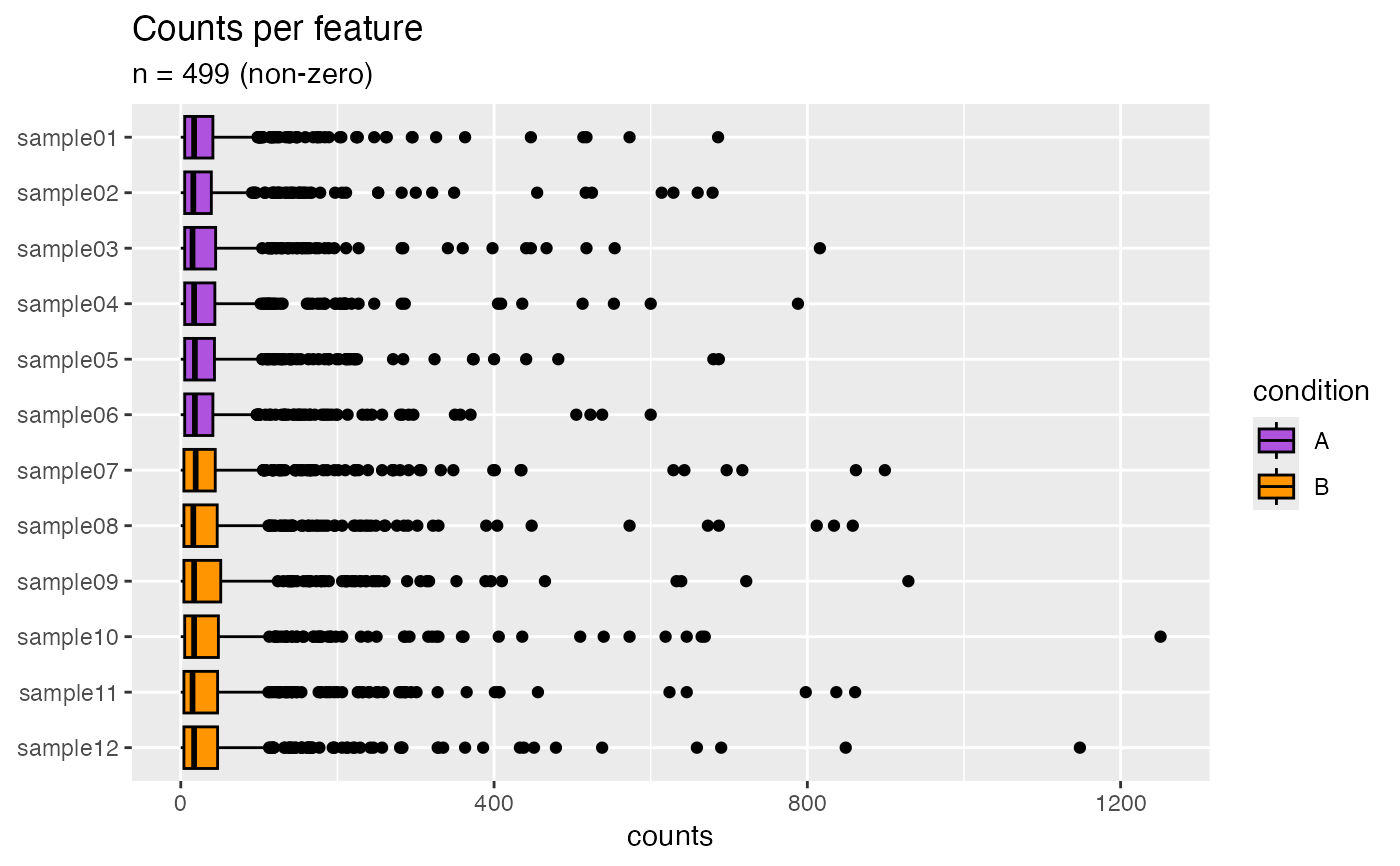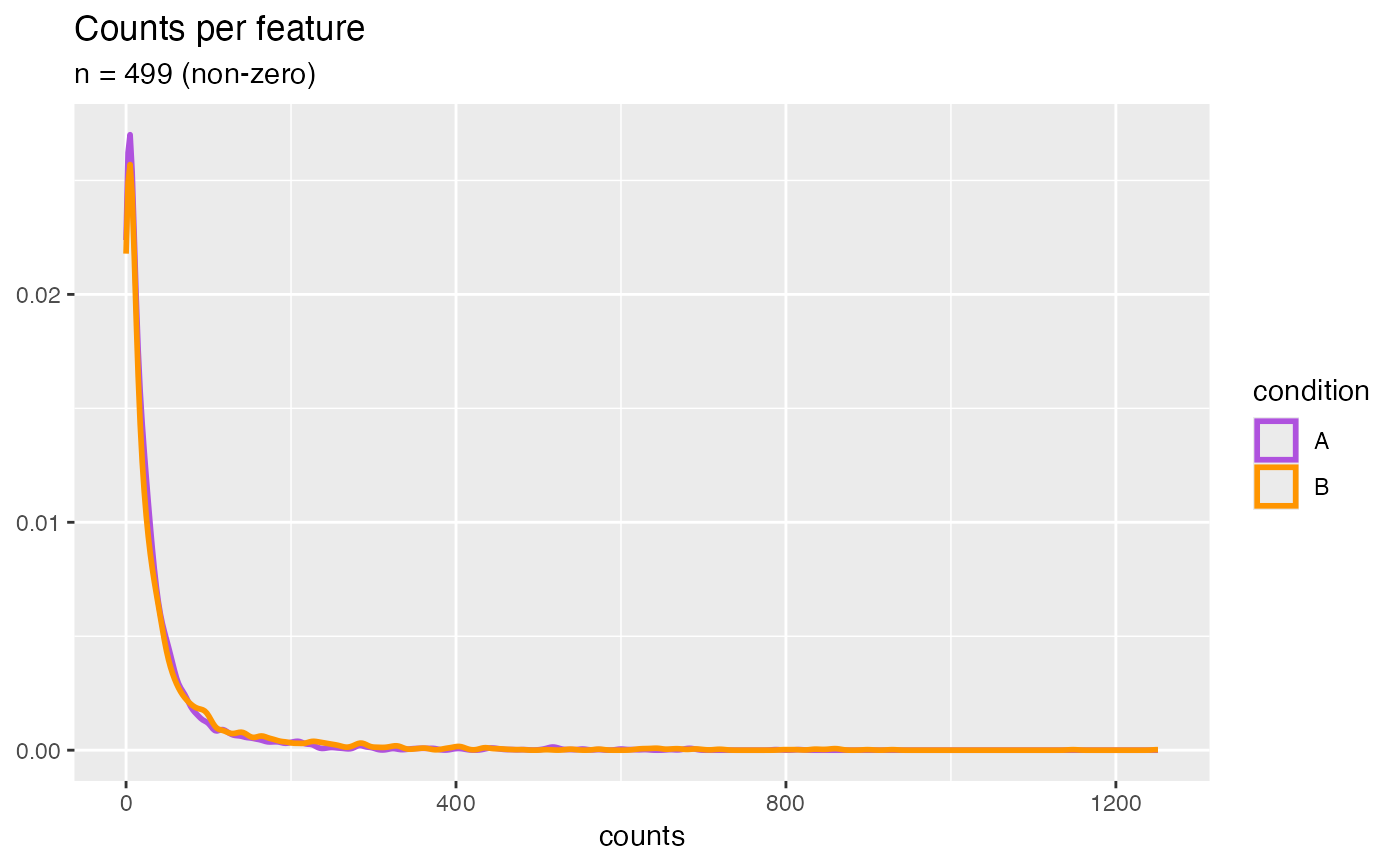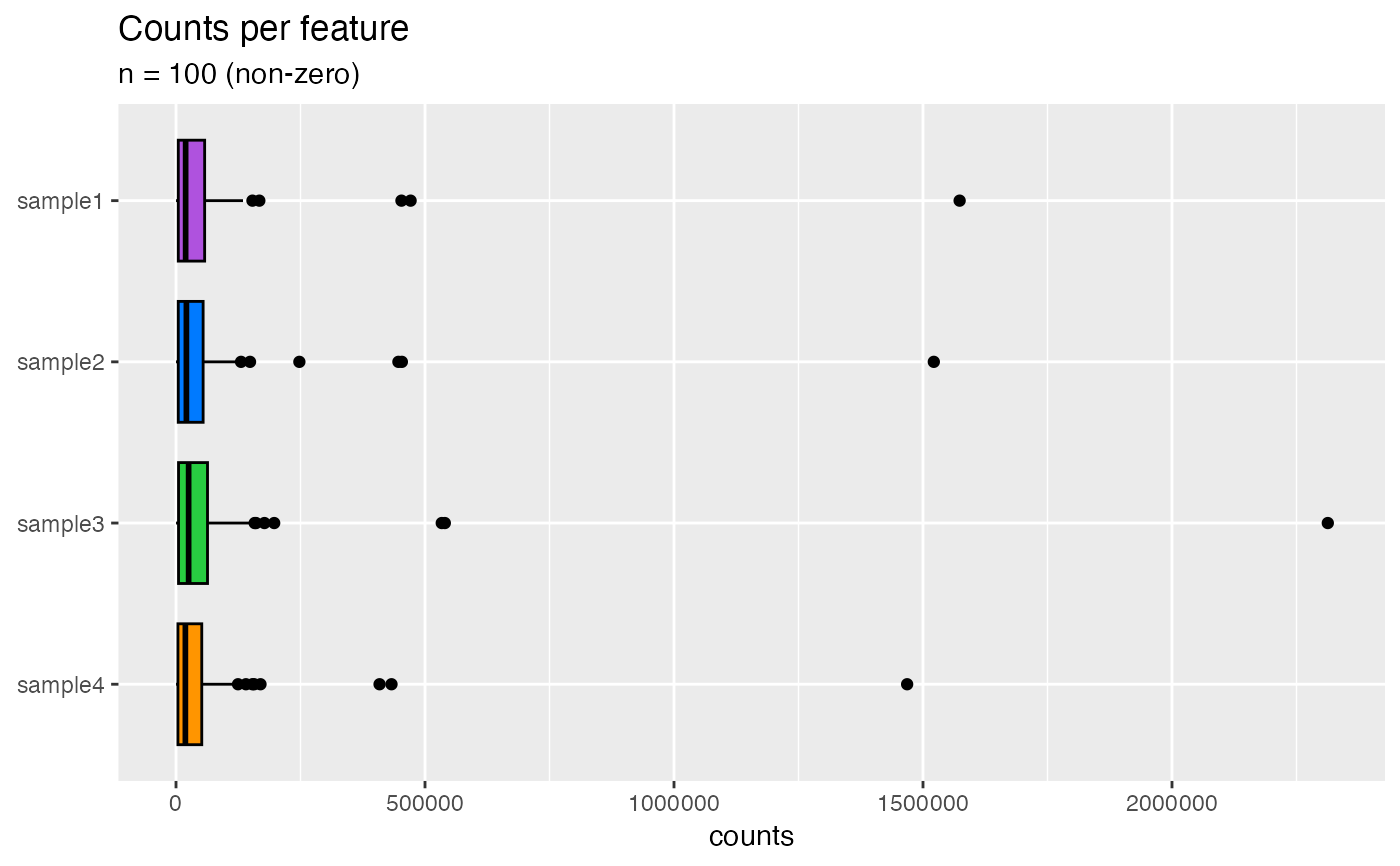Generally, we expect similar count spreads for all genes between samples unless the library sizes or total RNA expression are different.

## Usage

plotCountsPerFeature(object, ...)

# S4 method for SingleCellExperiment
plotCountsPerFeature(object, ...)

# S4 method for SummarizedExperiment
plotCountsPerFeature(
object,
assay = 1L,
interestingGroups = NULL,
geom = c("boxplot", "density", "jitter"),
trans = c("identity", "log2", "log10"),
labels = list(title = "Counts per feature", subtitle = NULL, sampleAxis = NULL,
countAxis = "counts"),
flip = getOption(x = "acid.flip", default = TRUE),
minMethod = c("absolute", "perRow")
)

## Arguments

object

Object.

...

assay

vector(1). Assay name or index position.

interestingGroups

character. Groups of interest to use for visualization. Corresponds to factors describing the columns of the object.

geom

character(1). Type of ggplot2 geometric object to use.

trans

character(1). Apply a log transformation (e.g. log2(x + 1L)) to the count matrix prior to melting, if desired. Use "identity" to return unmodified (default).

labels

list. ggplot2 labels. See ggplot2::labs() for details.

flip

logical(1). Flip x and y axes. Recommended for plots containing many samples.

minMethod

character(1). Only applies when min argument is numeric. Uses match.arg().

• absolute: Applies hard cutoff to counts column after the melt operation. This applies to all counts, not per feature.

• perRow: Applies cutoff per row (i.e. gene). Internally, rowSums() values are checked against this cutoff threshold prior to the melt operation.

## Value

ggplot.

## Functions

• plotCountsPerFeature(SingleCellExperiment): Applies aggregateCellsToSamples() calculation to summarize at sample level prior to plotting.
Passes ... to SummarizedExperiment method.

## Note

Updated 2023-08-11.

## Examples

data(
RangedSummarizedExperiment,
SingleCellExperiment_splatter,
package = "AcidTest"
)

## SummarizedExperiment ====
object <- RangedSummarizedExperiment
plotCountsPerFeature(object, geom = "boxplot")
#> ℹ Filtered zero count rows and columns:
#> - 499 / 500 rows (100%)
#> - 12 / 12 columns (100%)plotCountsPerFeature(object, geom = "density")
#> ℹ Filtered zero count rows and columns:
#> - 499 / 500 rows (100%)
#> - 12 / 12 columns (100%)## SingleCellExperiment ====
object <- SingleCellExperiment_splatter
plotCountsPerFeature(object)